# Looking through theApollonian Window

Apollonian Seminar, Room 356.
E-mail Jerzy Kocik: "jkocik at siu.edu"

Back to the main page

Pick a point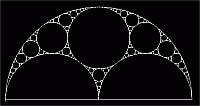BASIC STUFF    SOME GEOMETRY    ART
Here you find very basic construction and data

Pages produced with processing or other programs

Art related to Apollonian disk packing

 1. Pappus chain --- a 2200 year old geometric construction (interactive) at different sites: "Cut-the-Knot" 1, "Cut-the-Knot" 2, and by Thomas Schoch. 2. Descartes configuration -- an interactive applet. Try the same with movable centers (my humble use of Cinderella). 3. Apollonian gasket: Play with Apollonian gasket of the first dozen circles. 4. Template of the Apollonian Window (pdf) for printing. And here is the upper half only. 5. Draw your own Apollonian Window (recipe plus the data for the first seven hundred circles). 6. Spin structure of the Apolonian Window -- a poster, version 0.02 (Work in progress).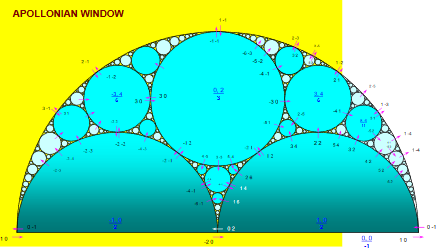7. Images. Some of my Povray figures inspired by the Apollonian window
 1. Symmetries. [interactive] Click to see various symmetries: Pappus threads, (a few thousand circles generated iteratively), only the external part (hyperbolic tasselation via triangles!), three-circle symmetries, and finally the galaxies as an extra bonus. 2. Satelites in the Apollonian Window. [interactive] For now Golden and Silver. For explanations, see "Lens sequences". 3. Chaos game [interactive] IFS done with Processing.js. (reproduced also at the top of this page). Click on the picture to start a new process) Here is the math behind the process. 4. Dedekind tessellation [interactive]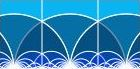See also some pictures. 5. Apollonian Galaxy. See also its center, a big picture, and a third-kind encounter (click on the corresponding image).
 1. A beautifull image of the regular Apollonian gasket by Jos Leys See more here. The movie Dimensions, which he made together with Etienne Ghys and Aurélien Alvarez, won the "Prix d'Alembert" award from the French Mathematical Society. Processing implementation of Kate Stange's idea of tracing orbits of $$\hbox{SL}(2,\mathbb Z[i])$$ acting on circles.My texts related to the Apollonian disk packing
and Descartes configurations

(Sources of the basic definitions or theorems are marked with )

Geometry

Related to the tangency spinors

1A. "A Theorem..." Shows the correspondence of disks (circles) and the Minkowski space. Terms "co-curvature", "reduced coordinates, "circle symbols" are introduced. Descartes theorem is a special case of the theorem proved here.
1B. "Proof..." is a succinct version of the above.
The rest are various applications.
Except for "On Diophantine... which stands alone.
"Apollonian Window" was named so in "Clifford..." in the other column.

Fibonacci numbers and Ford circles (8 pages, arXiv:2003.00852) An amusing connection between Ford circles, Fibonacci numbers, and golden ratio is shown. Namely, certain tangency points of Ford circles are concyclic and involve Fibonacci numbers. They form four circles that cut the x-axis at points related to the golden ratio.

Apollonian depth and the accidental fractal (35 pages, arXiv:2002.04135) The depth function of three numbers representing curvatures of three mutually tangent circles is introduced. Its 2D plot leads to a partition of the moduli space of the triples of mutually tangent circles/disks that is unexpectedly a beautiful fractal, the general form of which resembles that of an Apollonian disk packing, except that it consists of ellipses instead of circles.

A note on unbounded Apollonian disk packings (10 pages, arXiv:1910.05924) A construction and algebraic characterization of two unbounded Apollonian Disk packings in the plane and the half-plane are presented. Both turn out to involve the golden ratio.

On the Dedekind tessellation (16 pages, arXiv:1912.05768) The Dedekind tessellation is usually viewed as a system of ideal triangles obtained through the action of PSL(2,$$\mathbb Z)$$ in the upper half-plane. But here it is viewed it rather as a system of circles and give their complete characterization with the help of the Lorentz group acting in the Minkowski space of circles. Interesting interplay of geometry, group theory and number theory. Plus a simple algorithm for drawing the Dedekind tessellation. Plus Fibonacci numbers.

Skein relations for spin networks, modified, Journal of Knot Theory and Its Ramifications 27 (7) 2018 (21 pages, arXiv:1807.07244) Although the main matter is a skein relation for spin networks, the last sections consider Apollonian disk packings as a source of spin networks.

The Koide Lepton Mass Formula and Geometry of Circle Configurations (3 pages, arXiv:1201.2067) A remarkable formal similarity between Koide's Lepton mass formula and a generalized Descartes circle formula is reported. Also, a similar formula for quarks is proposed.

Proof of Descartes circle formula and its generalization clarified ( arXiv:1910.09174). A succict, to-the-point, version of the derivation of the (generalized) Descartes circle theorem, first presented in the paper below.

A theorem on circle configurations (arXiv:0706.0372). A generalization of the Descartes circle theorem to quite arbitrary configurations. The correspondence between circles in a plane and vectors in Minkowski space is utilized. The "extended Descartes theorem" in "Beyond the Descartes theorem" by Lagarias at al. (see below in Readings) is a special case for circles that are tangent.

Lens sequences (33 pages, arXiv:0710.3226). About sequences of curvatures of chains of circles inscribed in lenses, i.e., intersections of two overlapping disks of the same radius. Lots of intriguing properties. Such lens sequences appear in the Apollonian Window. Golden ratio and Fibonacci and Lucas numbers appear through them.

On a Diophantine equation that generates all integral Apollonian disk packings ISNR Geometry, 17 2007 pp. 71-93. A simple purely geometric derivation of the Diophantine equation and algorithm to generate all integral Apollonian packings. Includes some remarks on Pythagorean triples occurring in the packings.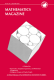Golden window (Mathematics Magazine, 83 Dec 2010, pp. 384-90). A very light text about a window built with design that conceals the golden ratio and its various powers. The cover features a figure based on this article.

1. "Clifford..." introduces the idea of tangency spinor (without the term "tangency").
2. "Spinors..." contains the key theorems on the tangency spinors. Does not require reading 1.
3A. "Apollonian coronas..." and 3B. "Tessellations..." are parallel and use the facts proved in 2. They also contain a short account of 2.

Spinors, lattices, and classification of integral Apollonian disk packings. (35 pages, arXiv:2001.05866) Two integral tangency spinors (2-vectors) are shown to define integral Descartes configuration, and an integral Apolloian disk packing. Supprizingly, the result may be restated in terms of the Pauli spinors. Other keywords: celestial sphere, hyperbolic plane, Hopf fibration,

Tessellations and Descartes disk configurations (12 pages, arXiv:1910.05919) An intriguing correspondence between certain finite planar tessellations and the Descartes circle arrangements is presented. This correspondence may be viewed as a visualization of the spinor structure underlying Descartes circles.

Apollonian coronas and a new zeta function (19 pages, arXiv:1909.09941) A formula for the area of disks tangent to a given disk in an Apollonian disk packing (corona) in terms of a certain novel arithmetic Zeta function is found. The idea is based on "tangency spinors" defined for pairs of tangent disks.

Spinors and the Descartes configuration of circles , (21 pages, arXiv:1909.06994) Tangency spinors are defined for pairs of tangent disks in the Euclidean plane. A number of theorems are proved, one of which may be interpreted as a "square root of Descartes Theorem". In any Apollonian disk packing, spinors form a network. In the Apollonian Window, a special case of Apollonian disk packing, all spinors are integral.

Clifford Algebras and Euclid's parameterization of Pythagorean Triples Advances in Applied Clifford Algebras (Mathematical Structures), 17 2007 pp. 71-93). Why Euclid's parameters of pythagorean triples are "spinors". You may find here also formulas for parameterization of Pythagorean quadruples, hexads and decuples (using quaternions and octonions) as well as a geometric interpretation of Hall matrices in the context of the Apollonian Window.##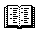Readings

Jeffrey Lagarias at al.: Beyond the Descartes configuration -- the generalization of Descartes theorem on four circles (2002).

Bo Soderberg: Apollonian tiling, the Lorentz group, and regular trees -- a physicist on the correspondence between the circles and Minkowski space-time, and much more. Rich but very parsimonious in giving credits to others (1992).

Donald Coxeter: The Problem of Apollonius -- (1968)

J.B. Wilker: Four proofs of a generalization of the Descartes circle theorem (1969)

Peter Sarnak: Integral Apollonian Packings Am Math Montly (Apr 2011)

More papers on Apollonian gaskets by Lagarias at al.:

More papers of some relevance:

Pfiefer and Van Hook: Circles, Vectors and Linear algebra -- a very simple introduction to the concept of Minkowski space as parameter space of circles in plane (1993)
W.S. Brown: Kiss Precise -- an old paper on tangent spheres (1969)

Kenneth Stephenson: Circle Packing: A Mathematical Tale, Notices of AMS, Vol. 50 (11) Dec 2003, pp. 1376--1388.

M. Borkovec, W. De Paris, and R. Peikert: The fractal dimension of the Apollonian sphere packing

XKCD: What if?...

Vladimir Arnold: On Teaching Mathematics, an extended text of the address at the discussion on teaching of mathematics in Palais de D'ecouverte in Paris on 7 March 1997. (Some have marked it as "controversial").##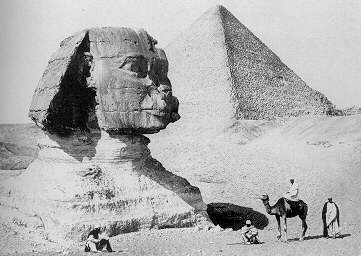Places

The On-Line Encyclopedia of Integer Sequences --- maintained by N. J. A. Sloane.Related sites:

Tangencies: Three Tangent Circles -- from Geometry Junkyard
Soddy Circles -- from Wolfram MathWorld
Apollonian Gasket -- from Wolfram MathWorld
Apollony fractal -- by Paul Bourke
Apollonian Gasket -- from Interactive Mathematics Miscellany and Puzzles
Apollonian Gasket -- Wolfram Demonstrations Project